Next: Calculation of Mean Values Up: Applications of Statistical Thermodynamics Previous: Spin-1/2 Paramagnetism

# System with Specified Mean Energy

Consider an isolated system,, that consists of a fixed number of particles contained in a given volume. Suppose that the the only available information about the system's energy is its mean value,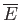. This might be the case, for example, if the system is brought to some final macrostate as a result of interaction with other macroscopic systems. Here, the measurement of the macroscopic work done on the system, or the heat that it absorbs, during this process does not tell us about the energy of each system in the corresponding ensemble, but, instead, only provides information about the mean energy of the final macrostate of.

A system,, with specified mean energy,, is also described by a canonical distribution. For, if such a system were placed in thermal contact with a heat reservoir held at some temperature,''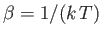, then the mean energy of the system would be determined. Thus, an appropriate choice of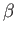would guarantee that the mean energy of the system had the specific value.

We can give a more direct proof of the previous assertion. Let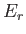be the energy of systemin some state. Suppose that the statistical ensemble consists of a very large number,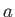, of such systems,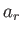of which are in state. Thus, the information available to us is that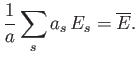(7.27)

Hence, it follows that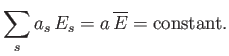(7.28)

This implies that the situation is equivalent to one in which a fixed amount of energy,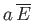, is to be distributed over all the systems in the ensemble, such that each system is equally likely to be in any one state. If a system in the ensemble is in statethen the remaining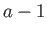systems must then have a combined energy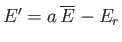. Thesesystems are distributed over some very large number,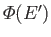, of accessible states. In other words, if the one system under consideration is in statethen the remainingsystems are distributed with equal probability in any of the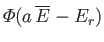states accessible to them. Because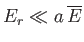, the mathematical problem here is exactly the same as that considered in Section 7.2, where we dealt with a system in thermal contact with a heat bath. In this case, the role of the heat bath is played by the totality of all the other systems in the ensemble. Accordingly, we again get the canonical distribution,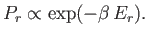(7.29)

The parameter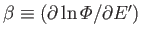does not here have any immediate physical significance in terms of the temperature of a real heat bath. Instead, it is to be determined by the condition that the mean energy calculated using the distribution has the specified value. In other words,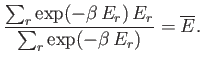(7.30)

In conclusion, if one is dealing with a system in contact with a heat bath held at temperature''then the canonical distribution, (7.29), is valid, and the mean energy,, can be calculated from Equation (7.30), using the known value of. On the other hand, if one is dealing with a system of specified mean energy,, then the canonical distribution, (7.29), is again valid, but the parameteris to be calculated using Equation (7.30) and the known value of.Next: Calculation of Mean Values Up: Applications of Statistical Thermodynamics Previous: Spin-1/2 Paramagnetism
Richard Fitzpatrick 2016-01-25